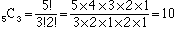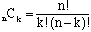Subject: odds and other Name: lucy Who is asking: Student Level: Secondary Question: If the odds are 1/15 of winning, what is the probability? if c 5! 3! How does it equal 10? Hi Lucy, We usually write odds as a ratio rather than a fraction. In your example I would say that "the odds are 1:15 of winning". To me this says that "in the long run" for every 16 times you play the game, you win once and lose 15 times. To say this using probability, the prabability that you win is 1/16. To take another example, if the odds of winning were 5:3 then "in the long run" for every 8 times you play, you win 5 times and lose three times. Hence the probability of winning is 5/8. In your second question I assume that you are asking about  5C3, that is the number of combinations of 5 things taken 3 at a time. The expression for 5C3 isFor any integers n and k, with k at most n, isCheers, Harley Go to Math Central